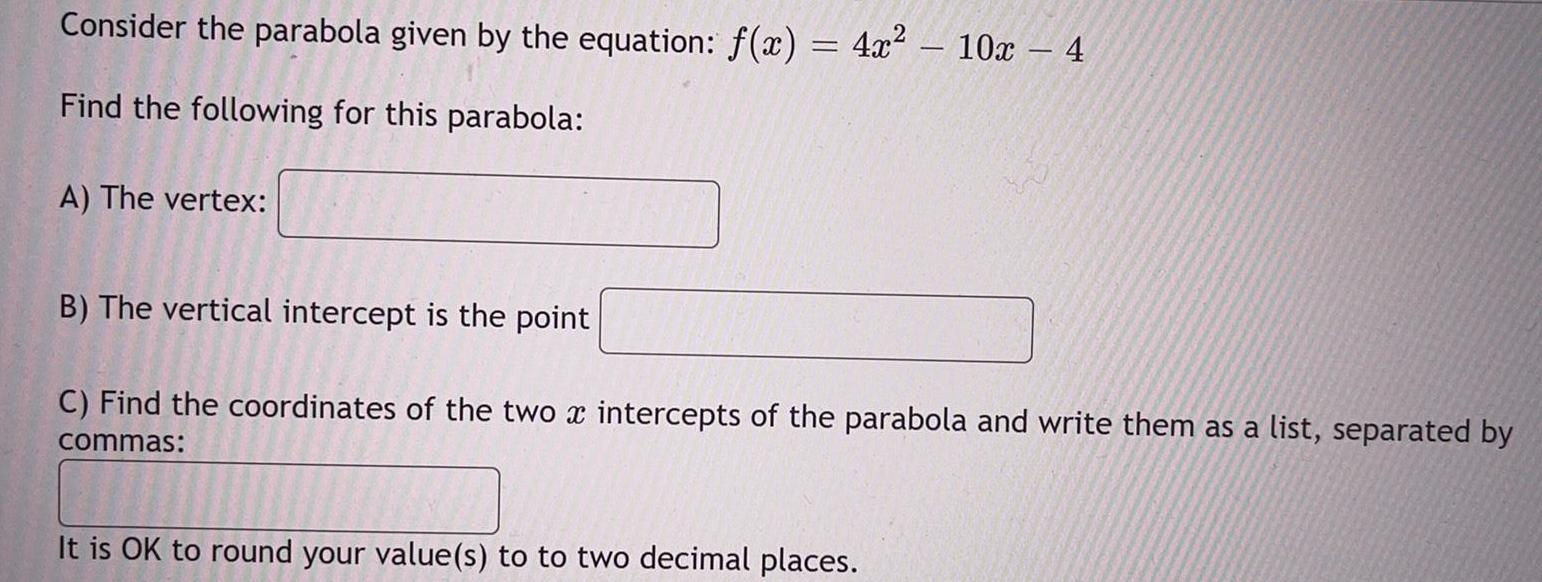Question:

# Consider the parabola given by the equation: f(x) = 4x²

Last updated: 8/26/2022Consider the parabola given by the equation: f(x) = 4x² – 10x-4 Find the following for this parabola: A) The vertex: B) The vertical intercept is the point C) Find the coordinates of the two x intercepts of the parabola and write them as a list, separated by commas: